# Big Ideas Math 8th Grade Chapter 2: Transformations Chapter Exam

Exam Instructions:

Choose your answers to the questions and click 'Next' to see the next set of questions. You can skip questions if you would like and come back to them later with the yellow "Go To First Skipped Question" button. When you have completed the practice exam, a green submit button will appear. Click it to see your results. Good luck!

### Page 1

#### Question 3 3. Which of the following rectangles represents a scale factor of 3 from the rectangle pictured below?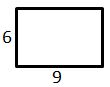#### Question 4 4. Which answer choice is similar to the triangle pictured below?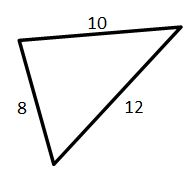#### Question 5 5. Josh claims that these triangles are similar by SSS. Do you agree or disagree with Josh?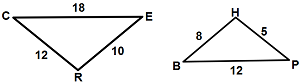### Page 2

#### Question 6 6. If the two triangles pictured below are similar, then what is x?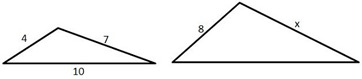#### Question 7 7. Determine if triangle VEZ is similar to triangle MAG.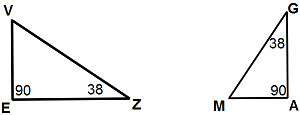#### Question 8 8. If the two triangles pictured below are congruent, then which angles are the same?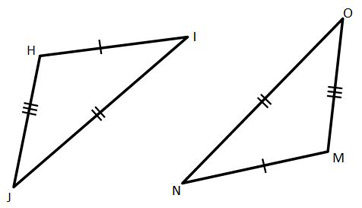#### Question 10 10. Mary claims that the triangles below are similar by AA. Do you agree or disagree with Mary?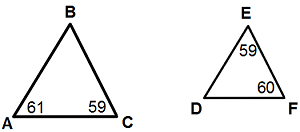### Page 3

#### Question 11 11. What is the scale factor from the smaller rectangle to the larger rectangle pictured below?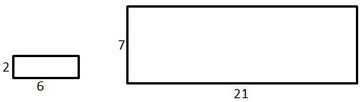#### Question 12 12. What is the mathematical notation for the translation shown below? (The red box is the original image, the blue box the final)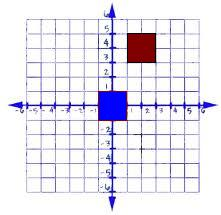#### Question 14 14. If triangle MNO is congruent to triangle PQR, then what is the measure of angle x?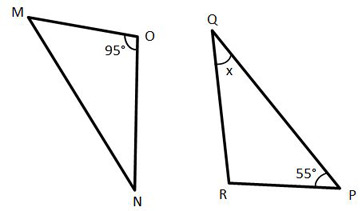#### Question 15 15. In the similar rectangles pictured below, what is y?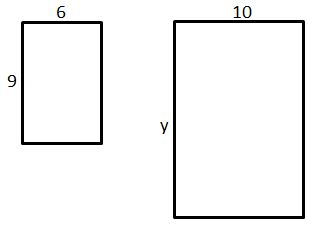### Page 4

#### Question 16 16. Conclude whether triangle BEN is similar to triangle MAT.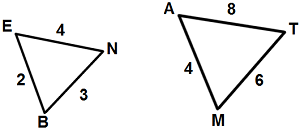#### Question 17 17. Is triangle ABC similar to triangle DEF?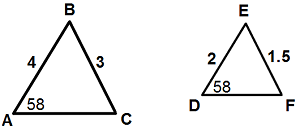#### Question 19 19. If we apply a scale factor of 4 to the triangular prism below, what will the volume of the new prism be?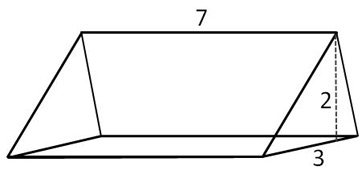#### Big Ideas Math 8th Grade Chapter 2: Transformations Chapter Exam Instructions

Choose your answers to the questions and click 'Next' to see the next set of questions. You can skip questions if you would like and come back to them later with the yellow "Go To First Skipped Question" button. When you have completed the practice exam, a green submit button will appear. Click it to see your results. Good luck!

Support